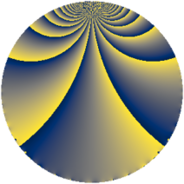# Properties

 Label 585.1.oLevel $585$ Weight $1$ Character orbit 585.o Rep. character $\chi_{585}(298,\cdot)$ Character field $\Q(\zeta_{4})$ Dimension $8$ Newform subspaces $1$ Sturm bound $84$ Trace bound $0$

# Related objects

## Defining parameters

 Level: $$N$$ $$=$$ $$585 = 3^{2} \cdot 5 \cdot 13$$ Weight: $$k$$ $$=$$ $$1$$ Character orbit: $$[\chi]$$ $$=$$ 585.o (of order $$4$$ and degree $$2$$) Character conductor: $$\operatorname{cond}(\chi)$$ $$=$$ $$65$$ Character field: $$\Q(i)$$ Newform subspaces: $$1$$ Sturm bound: $$84$$ Trace bound: $$0$$

## Dimensions

The following table gives the dimensions of various subspaces of $$M_{1}(585, [\chi])$$.

Total New Old
Modular forms 24 12 12
Cusp forms 8 8 0
Eisenstein series 16 4 12

The following table gives the dimensions of subspaces with specified projective image type.

$$D_n$$ $$A_4$$ $$S_4$$ $$A_5$$
Dimension 8 0 0 0

## Trace form

 $$8 q + O(q^{10})$$ $$8 q - 8 q^{10} - 8 q^{16} + 8 q^{22} + 8 q^{40} - 8 q^{43} - 8 q^{52} - 8 q^{82} + 16 q^{88} + O(q^{100})$$

## Decomposition of $$S_{1}^{\mathrm{new}}(585, [\chi])$$ into newform subspaces

Label Dim $A$ Field Image CM RM Traces $q$-expansion
$a_{2}$ $a_{3}$ $a_{5}$ $a_{7}$
585.1.o.a $8$ $0.292$ $$\Q(\zeta_{16})$$ $D_{8}$ $$\Q(\sqrt{-39})$$ None $$0$$ $$0$$ $$0$$ $$0$$ $$q+(\zeta_{16}^{5}-\zeta_{16}^{7})q^{2}+(-\zeta_{16}^{2}+\zeta_{16}^{4}+\cdots)q^{4}+\cdots$$